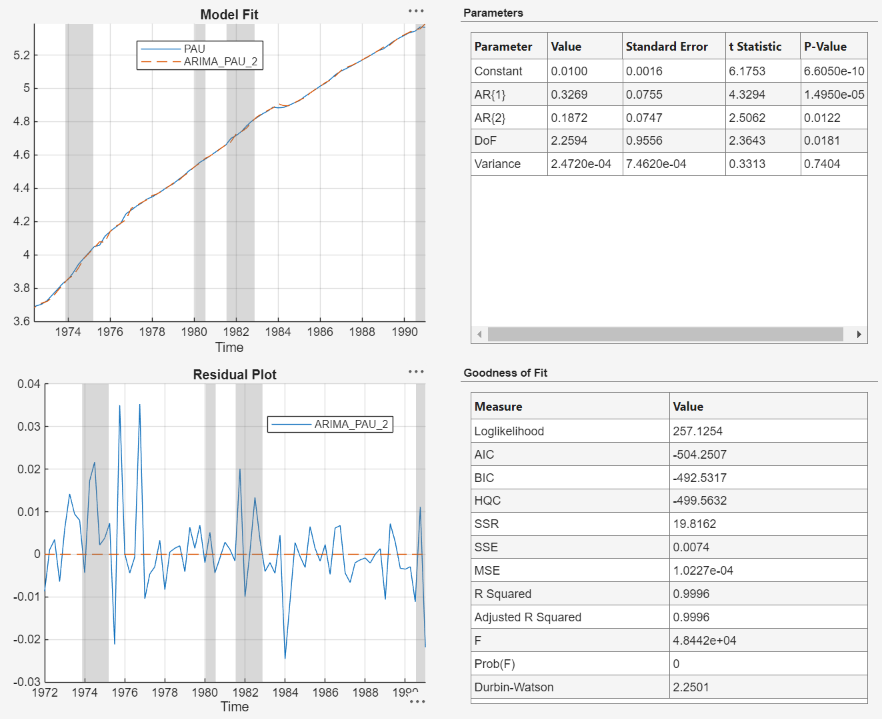Main Content

## Specify t Innovation Distribution Using Econometric Modeler App

This example shows how to specify a t innovation distribution for an ARIMA model by using the Econometric Modeler app. The example also shows how to fit the model to data. The data set, which is stored in `Data_JAustralian.mat`, contains the log quarterly Australian Consumer Price Index (CPI) measured from 1972 and 1991, among other time series.

### Import Data into Econometric Modeler

At the command line, load the `Data_JAustralian.mat` data set.

`load Data_JAustralian`

Convert the table `DataTable` to a timetable:

1. Clear the row names of `DataTable`.

2. Convert the sampling times to a `datetime` vector.

3. Convert the table to a timetable by associating the rows with the sampling times in `dates`.

```DataTable.Properties.RowNames = {}; dates = datetime(dates,'ConvertFrom','datenum',... 'Format','ddMMMyyyy','Locale','en_US'); DataTable = table2timetable(DataTable,'RowTimes',dates);```

At the command line, open the Econometric Modeler app.

`econometricModeler`

Alternatively, open the app from the apps gallery (see Econometric Modeler).

Import `DataTable` into the app:

1. On the Econometric Modeler tab, in the Import section, click.

2. In the Import Data dialog box, in the Import? column, select the check box for the `DataTable` variable.

3. Click .

The variables, including `PAU`, appear in the Time Series pane, and a time series plot containing all the series appears in the Time Series Plot(EXCH) figure window.

Create a time series plot of `PAU` by double-clicking `PAU` in the Time Series pane.### Specify and Estimate ARIMA Model

Estimate an ARIMA(2,1,0) model for the log quarterly Australian CPI. Specify a t innovation distribution. (For details, see Implement Box-Jenkins Model Selection and Estimation Using Econometric Modeler App and Perform ARIMA Model Residual Diagnostics Using Econometric Modeler App.)

1. In the Time Series pane, select the `PAU` time series.

2. On the Econometric Modeler tab, in the Models section, click ARIMA.

3. In the ARIMA Model Parameters dialog box, on the Lag Order tab:

1. Set the Degree of Integration to `1`.

2. Set the Autoregressive Order to `2`.

3. Click the button, then select `t`.4. Click .

The model variable `ARIMA_PAU` appears in the Models pane, its value appears in the Preview pane, and its estimation summary appears in the Model Summary(ARIMA_PAU) document.The app estimates the t innovation degrees of freedom (DoF) along with the model coefficients and variance.

Download ebook